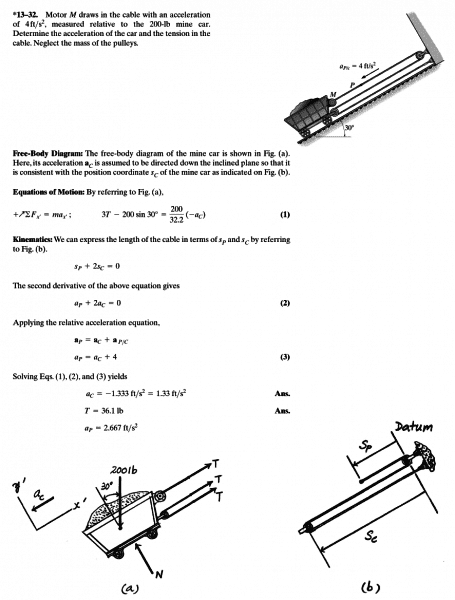# Trying to understand the solution given

• issacnewton

#### issacnewton

Hello, following is the mechanics problem involving pulley from an engineering mechanics book. I am trying to understand the solution given. As the car is being pulled, upward of the incline is assumed to be positive x direction. But when they apply the relative acceleration equation, in eq 3, the acceleration of the point P with respect to the car is entered as +4. Since the acceleration of the point P with respect to the car is acting downward the incline, this should be entered as -4 in equation 3. What people here think ? I have attached the problem and its solution as the PNG image file.thank you

Read it more carefully. It says the position and acceleration of the car are measured down the plane.

Hello haruspex, sorry for late reply. But where is the statement which says that position and acceleration are measured down the plane ? If you look at the picture of the axes given in the solution, positive x-axis is point upward the incline...

Look at the direction of the arrows in diagram (b).

Hello haruspex, sorry for late reply. But where is the statement which says that position and acceleration are measured down the plane ? If you look at the picture of the axes given in the solution, positive x-axis is point upward the incline...
Second sentence in the paragraph headed "free body diagram"

yes, I see now. thanks both of you.